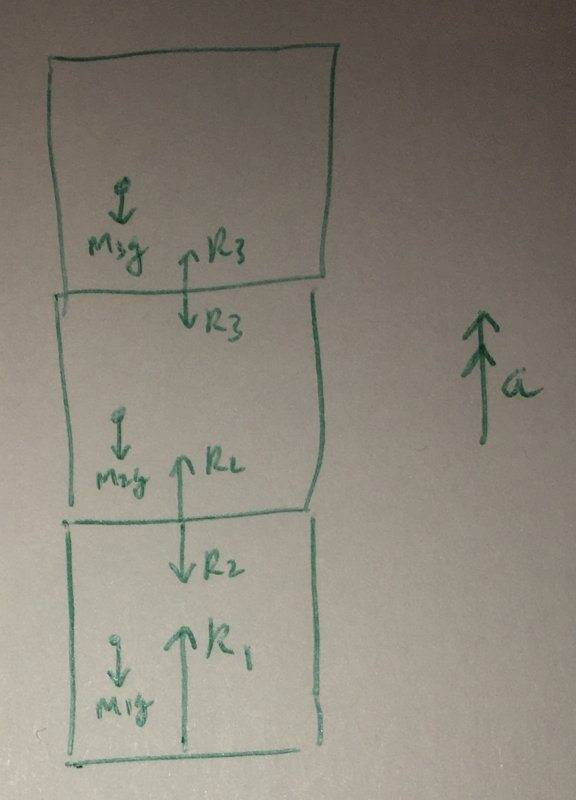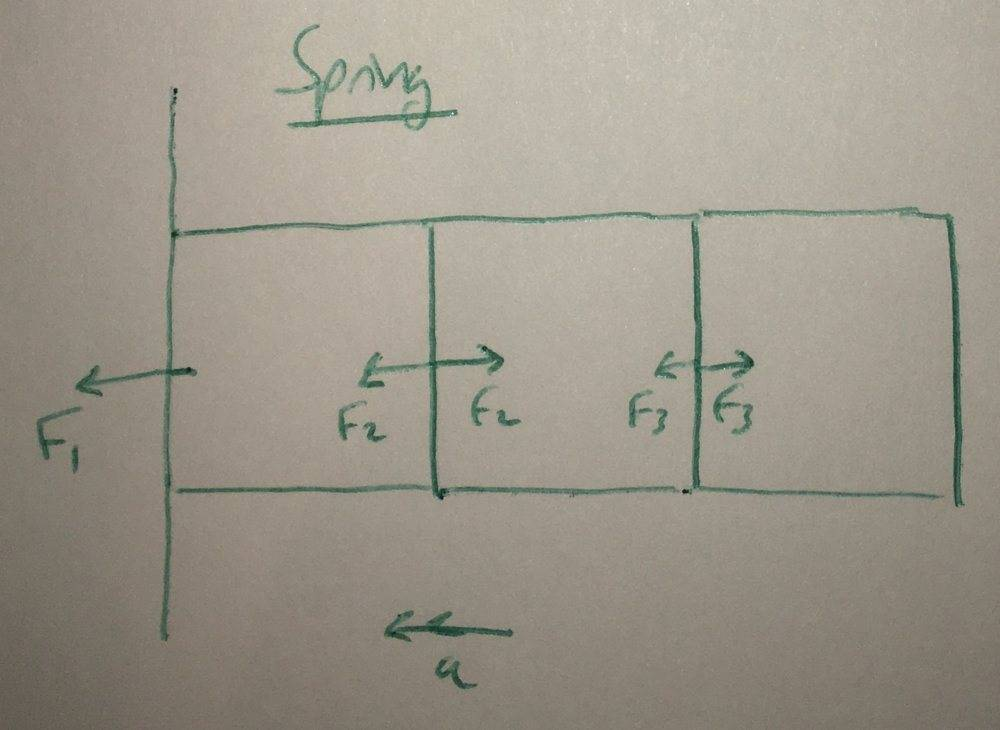# How can a reaction force do work on someone who is jumping?

• etotheipi

#### etotheipiLet $R_{1}$ be the reaction force on the jumper from the ground. Assuming this is larger than the combined mass of the 3 sections of human, if we consider the entire system we will get an upward acceleration (a is labelled as the acceleration of the COM; it will of course vary from the bottom to the top of the system).

For the top block on its own, the force $R_{3}$ evidently does work causing the top block's KE to increase. The same is true for the middle block as the work done by the lower reaction force is greater than the negative work done by the top reaction force (and weight) acting on it.

However, considering the entire system as a whole, the work done by the pair reaction forces should exactly cancel and the only force remaining is the force $R_{1}$ for which the point of application is stationary, so this force does no work. However, the kinetic energy of the system must increase so work has to have been done. Where does this occur?

This stemmed from a related question I had concerning springs; if we stretch a massive spring with one end attached to a wall, and consider the entire spring as accelerating back toward the wall when we release it, the net force acting on the spring is the reaction force from the wall which doesn't move! Please see the following diagram:The force $F_{1}$ obviously results in an acceleration, yet does no work! How does this work? Thank you very much in advance!

However, the kinetic energy of the system must increase so work has to have been done.
The chemical potential energy of the system is decreased by the same amount so no work was done

•etotheipi
The chemical potential energy of the system is decreased by the same amount so no work was done

This sounds like it is right, though I don't really understand the mechanism. If work is defined as the change in kinetic energy of a body, and the body is definitely gaining KE, then surely some work is done by an upward force. I suppose the forces produced my muscles might be responsible, as the point of application definitely changes position, which is in effect the conversion of CPE you mentioned.

However, what about the spring scenario? From a NII perspective the reaction force from the wall causes acceleration, but in this case there are no other internal forces other than all of the internal spring forces which, when considering the system as a whole, should do equal amounts of positive and negative work which sum to zero.

but in this case there are no other internal forces other than all of the internal spring forces which, when considering the system as a whole, should do equal amounts of positive and negative work which sum to zero.
The forces on the two ends of a spring can most certainly produce non-zero total work.

The forces on the two ends of a spring can most certainly produce non-zero total work.

But if the free end of the spring is not attached to anything then isn't there no force acting at this end apart from that responsible for accelerating this "final segment" of spring, which is exactly canceled by another when considering the work done on the system as a whole (I'm thinking along the lines of $F_{3}$ in the second diagram, where both reaction forces undergo the same displacement).

Even if it was attached to a mass at the end, then wouldn't the force acting on the end of the spring be in the opposite direction to motion, which would actually result in negative work done whilst producing a positive change in KE?

But if the free end of the spring is not attached to anything
Then for things to make sense, the spring must be massive. And every portion of it will be moving and will have work done on it.

If work is defined as the change in kinetic energy of a body,
Work isn’t defined that way.

Work is defined one of two ways. In Newtonian mechanics it is a force times a displacement. In thermodynamics it is a transfer of energy by any mechanism besides heat.

With either definition the work is 0 in this scenario.

I think that you are thinking about the work energy theorem. It is not a definition, it is a theorem and it only applies for rigid bodies.

Work isn’t defined that way.

Work is defined one of two ways. In Newtonian mechanics it is a force times a displacement. In thermodynamics it is a transfer of energy by any mechanism besides heat.

With either definition the work is 0 in this scenario.

In what situations does the work energy theorem then apply?

In what situations does the work energy theorem then apply?
It only applies for rigid bodies.

•etotheipi
It only applies for rigid bodies.

I think this resolves it. In the case of the massive spring recoiling, it is definitely not a rigid body due to the deformation occurring, so W/E theorem does not apply and it does not matter that no work is done on the spring system for it to be accelerating. Whilst each small slice of the spring is a rigid body so we can definitely calculate $\Delta E_{k}$ for each bit.

In the case of the person jumping, I assume the force analysis is significantly more complicated (getting into how muscles apply forces etc.) so it is best to use another method such as considering the impulse the resultant of the reaction force & weight exerts on the jumper. It is instead the work done by muscles which results in the change in kinetic energy of the jumper.

Last edited by a moderator:
The change in kinetic energy of a system can be produced by the work of internal forces too. Review the work-energy theorem for a system of bodies with internal forces. It's the momentum that cannot be changed by internal forces but not so for KE.

•Delta2 and PeroK
Summary: Please see the diagram: assuming a person to be "3 blocks", I am unsure as to which force is doing work on the jumper.

The force F1F1F_{1} obviously results in an acceleration, yet does no work!
If the place that the force is applied doesn't move then no work is done. Why should that bother you? Work, along with a lot of other things, is frame dependent and in another frame the work would be non-zero.
People keep posting questions about 'Work On' and 'Work By'. Why? That is nothing to do with the Physics of a situation; it's just WORDS. If you stick with the Maths, you can always get consistent answers for these questions. isn't that enough?•etotheipi
Whilst each small slice of the spring is a rigid body so we can definitely calculate $\Delta E_{k}$ for each bit.
Be careful. If you add up (integrate) the work done on each slice by its neighbors, the total can be non-zero.

•sophiecentaur
Be careful. If you add up (integrate) the work done on each slice by its neighbors, the total can be non-zero.
+1+1+1 Every link in a chain passes on Energy but the FΔx's cancel out because the F's are in different directions but the Δx's are in the same direction. (that is when the chain is not accelerating; if the chain is accelerating, the F's are of different magnitude). Again the fog of 'on' and 'by' comes in and adds confusion.

+1+1+1 Every link in a chain passes on Energy but the FΔx's cancel out because the F's are in different directions but the Δx's are in the same direction. (that is when the chain is not accelerating; if the chain is accelerating, the F's are of different magnitude). Again the fog of 'on' and 'by' comes in and adds confusion.
We are not talking about a chain -- every link of which is rigid and has a fixed length. We are talking about a non-rigid spring.

We are not talking about a chain -- every link of which is rigid and has a fixed length. We are talking about a non-rigid spring.
I realize that but it demonstrates, yet again, the care that's needed when trying to work out the real situation. In the case of a spring, both Δx and F vary with position.

•jbriggs444